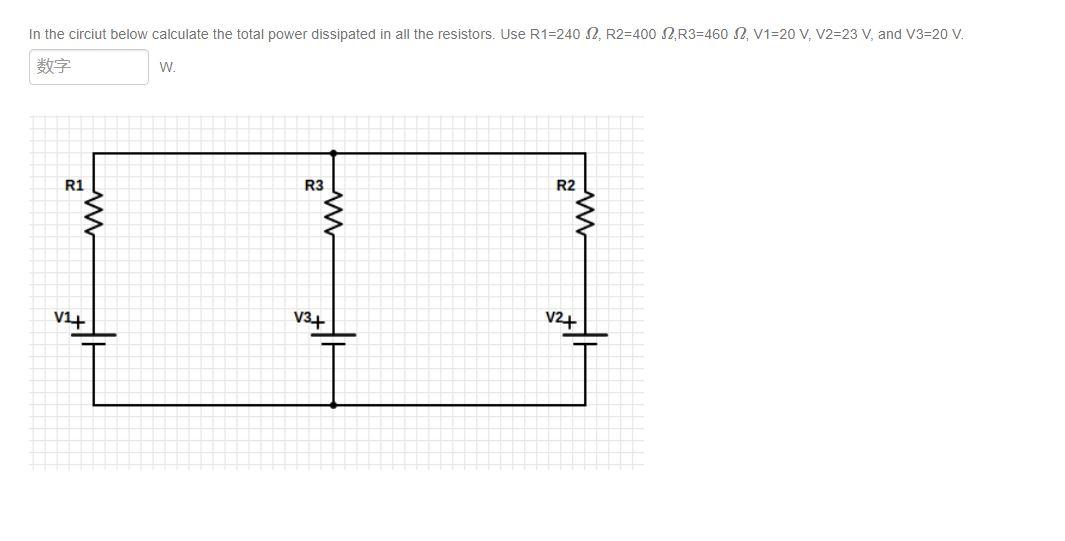# Question my answer is 0.036 w. but its incorrect In the circiut below calculate the total power dissipated in all the resistors. Use R1=240 2. R2=400 2, R3=460 2 V1=20 V, V2=23 V, and V3=20 V. 字 W. R1 R3 R2. w w V+ V34 V24PHUDAJ The Asker · Electrical Engineeringmy answer is 0.036 w. but its incorrect

Transcribed Image Text: In the circiut below calculate the total power dissipated in all the resistors. Use R1=240 2. R2=400 2, R3=460 2 V1=20 V, V2=23 V, and V3=20 V. 字 W. R1 R3 R2. w w V+ V34 V24
More
Transcribed Image Text: In the circiut below calculate the total power dissipated in all the resistors. Use R1=240 2. R2=400 2, R3=460 2 V1=20 V, V2=23 V, and V3=20 V. 字 W. R1 R3 R2. w w V+ V34 V24